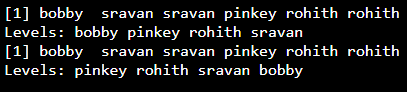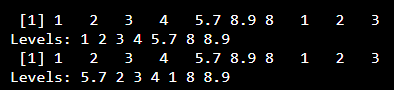Open in App
Not now

# How to change the order of levels of a factor in R?

• Last Updated : 23 May, 2021

In R programming language, factors are used to represent categorical data by uniquely identifying the elements from the given vector. It will return the levels of the unique elements when factor function is applied. In this article we are going to discuss how to change the levels of the factor.

We can get the levels of the vector using factor() function itself

Syntax: factor(vector)

Parameters:

• vector: vector with categorical data
• levels(): specifies the levels

Returns: vector elements with levels

If we want to change the order of the levels, we can specify the levels parameter in the factor() function

Syntax:

factor(vector,levels=c(elements))

levels parameter can accept the vector elements and within this, the order of levels can be passed as a vector.

Given below are various implementations for this approach:

Example:

## R

 `# Create a vector with elements``data =``c``(``"bobby"``, ``"sravan"``, ``"sravan"``, ``        ``"pinkey"``, ``"rohith"``,``"rohith"``)`` ` `#apply factor to vector to get ``# unique data``data=``factor``(data)`` ` `print``(data)`` ` `#change the order of the levels``# from the resultant data``ordered_data=``factor``(data,levels=``c``(``  ``'pinkey'``,``'rohith'``,``'sravan'``,``'bobby'``))`` ` `print``(ordered_data)`

Output:Example 2:

## R

 `# Create a vector with elements``data =``c``(1,2,3,4,5.7,8.9,8.0,1,2,3)`` ` `# apply factor to vector to get``# unique data``data=``factor``(data)``print``(data)`` ` `# change the order of the levels ``# from the resultant data``ordered_data=``factor``(data,levels=``c``(``  ``5.7,2,3,4,1,8,8.9))``print``(ordered_data)`

Output:My Personal Notes arrow_drop_up